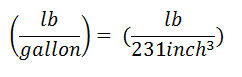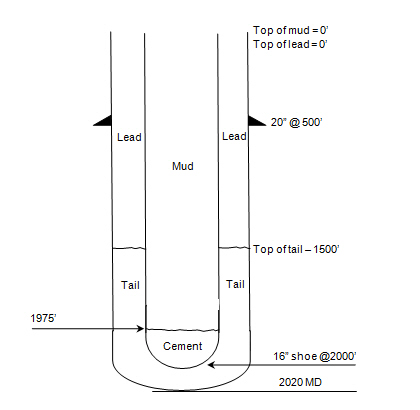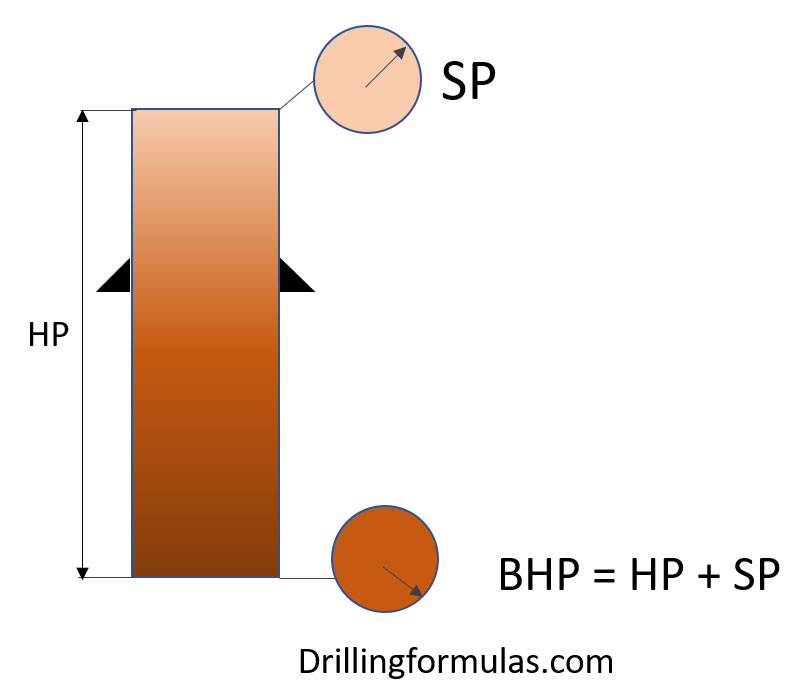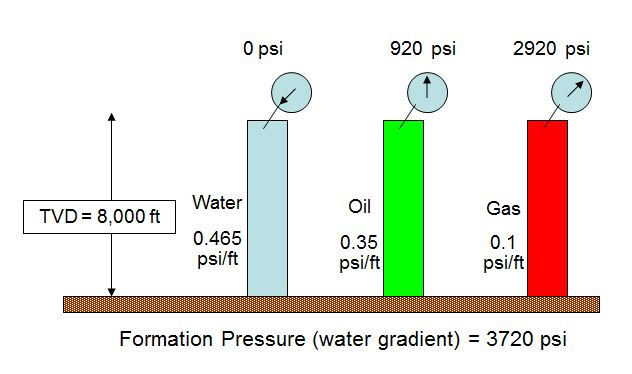## How does the 0.052 constant come from?

In the hydro-static pressure formula, if you use an oil field unit, you must see the hydro-static formula like this

Pressure, psi = 0.052 x Mud Weight (ppg) x TVD (ft)

I got the question asking about how the constant 0.052 is figured out.What is 0.052?

0.052 is a constant used for converting ppg-ft into pound per square inch (lb/in2)

Do you know how it comes from? Let’s get started

First of all, the unit conversions that you need to know are as follows:

1 gallon = 231 cubic inch (inch3)

1 ft = 12 inch

Convert density from ppg (pound per gallon) to lb per cubic inchBasic hydrostatic pressureThe constant can be determined by substituting the unit conversion.The constant is 0.051948 and if we round it up, it will be 0.052.

## How much pressure will we see at cement head in case of float shoe fail?

I still have the simple but interesting question about hydrostatic pressure which you can apply this calculation into drilling/cementing operation. The question I got about how much pressure we will see at cement head in case of float shoe fail.## Bottom Hole Pressure Relationship

This article will show you about bottom hole pressure relationship because this concept is very important for well control concept. The bottom hole pressure is a summation of all the pressure acting on the bottom hole.

Bottom Hole Pressure (BHP) = Surface Pressure (SP) + Hydrostatic Pressure (HP)

The image below demonstrates the relationship of bottom hole pressure.Note: BHP created by hydrostatic column of drilling fluid is the primary well control in drilling.

Looking more into details,

If BHP is more than FP (formation pressure), this situation is called “Overbalance”.

If BHP is equal to FP (formation pressure), this situation is called “Balance”.

If BHP is less than FP (formation pressure), this situation is called “Underbalance”.

For more understanding, please follow this example below it demonstrates the relationship of BHP, SP and HP.

Bottom Hole Pressure (BHP) = Surface Pressure (SP) + Hydrostatic Pressure (HP)We assume that formation pressure is normal pressure gradient of water (0.465 psi/ft) so formation pressure at 8000’ TVD = 8000 ft x 0.465 psi/ft = 3720 psi. Click here to learn how to calculate hydrostatic pressure in oilfield.

The first case: Hydrostatic column is water which is equal to formation pressure gradient so SP is equal to 0 psi

The second case: BHP is still be water gradient but fluid column is oil (0.35 psi/ft) which is lower density than water gradient (0.465 psi/ft). Therefore, in order to balance BHP, we need Surface Pressure (SP) of 920 psi (SP = 3720 – (0.35 x 8000)).

The third case: BHP is still be water gradient but fluid column is gas (0.1 psi/ft) which is even lower density than water gradient (0.465 psi/ft). Therefore, in order to balance BHP, we need Surface Pressure (SP) of 2,920 psi (SP = 3720 – (0.1 x 8000)).

According to the example, Surface Pressure (SP) will compensate the lack of hydrostatic pressure (HP) in order to balance formation pressure (FP).

References

Cormack, D. (2007). An introduction to well control calculations for drilling operations. 1st ed. Texas: Springer.

Crumpton, H. (2010). Well Control for Completions and Interventions. 1st ed. Texas: Gulf Publishing.

Grace, R. (2003). Blowout and well control handbook [recurso electrónico]. 1st ed. Paises Bajos: Gulf Professional Pub.

Grace, R. and Cudd, B. (1994). Advanced blowout & well control. 1st ed. Houston: Gulf Publishing Company.

Watson, D., Brittenham, T. and Moore, P. (2003). Advanced well control. 1st ed. Richardson, Tex.: Society of Petroleum Engineers.